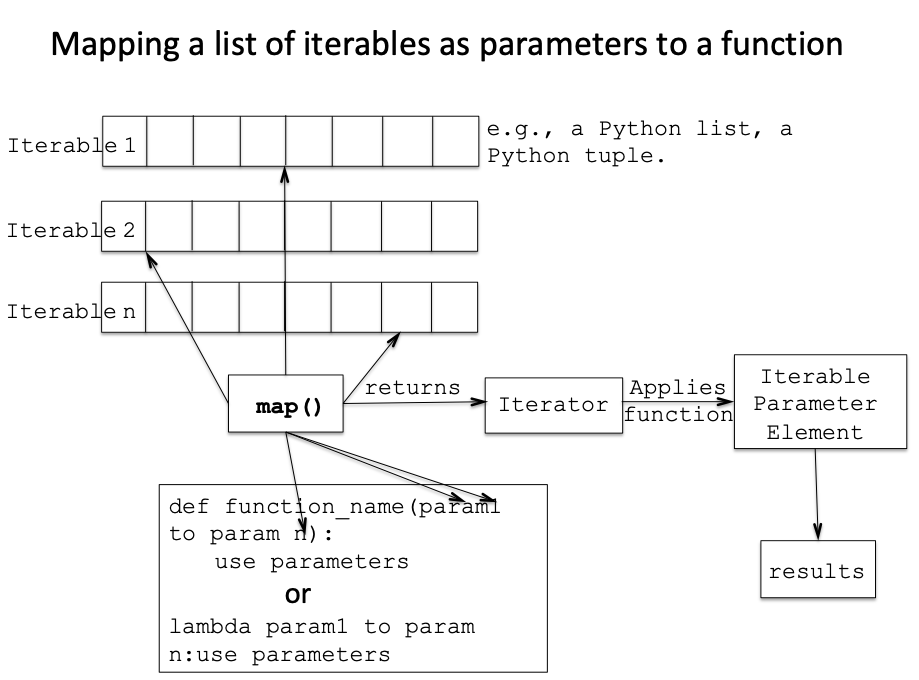# Map() Function In Python

## Overview:

• map() does a mapping between the following
• a function - either a regular function defined using def or an anonymous function defined using lambda
• the parameters of the lambda function
• one or more python iterables

and returns an iterator.

• The iterator when executed, for example using a, for loop – executes the function using its parameters. The parameters to the function are mapped to the python iterables passed to the map() function.
• Number of times the iterator is executed is equal to the count of elements present in the shortest iterable passed to the map() function.## Example – Using a lambda function with map():

 # Example Python program to apply lambda function to Python iterables # using the built-in function map multipliedBy2 = map(lambda x:x*x, [1,2,3,4,5]); print("The sequence of numbers multiplied by 2:"); for i in multipliedBy2:     print(i);

## Output:

 The sequence of numbers multiplied by 2: 1 4 9 16 25

## Example-Using a lambda function working on multiple iterables with map():

• It should be made sure that the parameter count of the lambda function and the iterables count of the map function to be the same. Else an error similar to TypeError: <lambda>() takes 1 positional argument but 3 were given will be raised.

 # Example Python program to apply a lambda function # to multiple Python iterables using the map() built-in function oddNumbers     = [1, 3, 5, 7, 9]; evenNumbers    = [2, 4, 6, 8, 10]; primeNumbers   = [2, 3, 5, 7];   # invoke the map() built-in function outputIterator = map(lambda x,y,z:x*y*z, oddNumbers, evenNumbers, primeNumbers);   # Print the return values of the lambda function by using the iterator for i in outputIterator:     print(i);

## Output:

 4 36 150 392